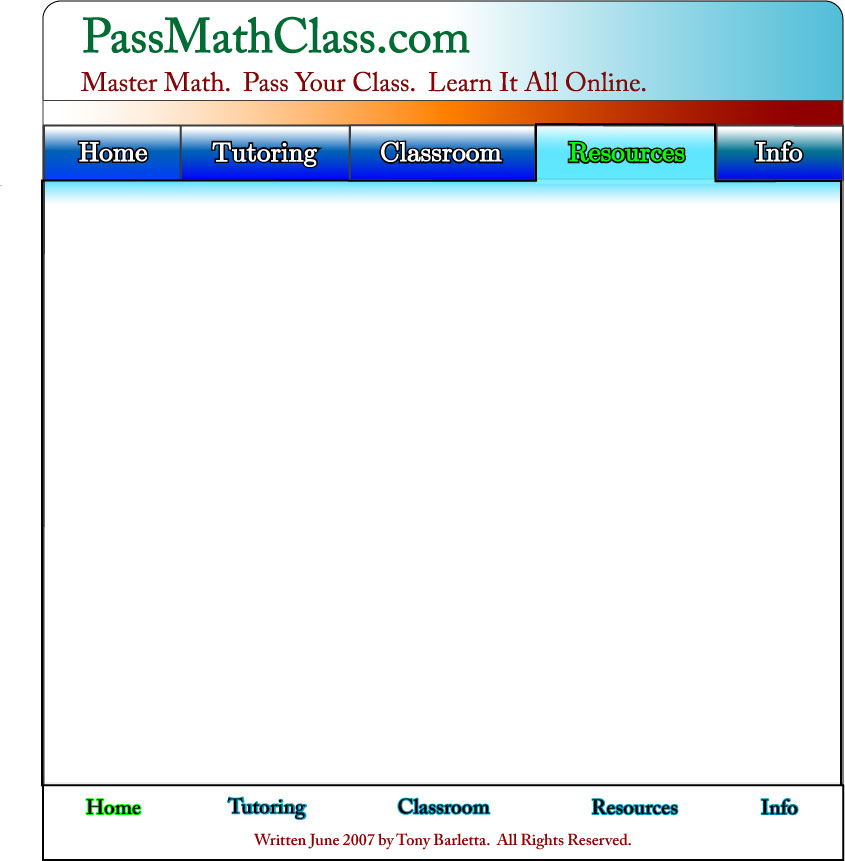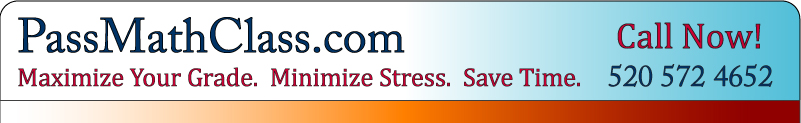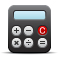This is a collection of free math resources. This material was written by the founders of Pass Math Class to not be like a textbook. We have seen many math textbooks that are great at rigorous proof and complex notation but terrible at actually explaining the material.

We have written these hoping that we can get straight to the important points, and help people actually learn. We are constantly adding new lessons and improving the existing ones, in an effort to make a truly complete resource for all students of mathematics. If you want to request a specific lesson, just let us know.

Feel free to go through all of the lessons, or just the ones you may need. And remember, we've got one-on-one tutoring AND drop-in math help.

We have left out the rigorous proofs and confusing vocabulary – we have kept the most simple essence of each concept.

Free Online Math LessonsFree Math Resources
Calculus
Pre-Algebra
Algebra
Pre-CalculusCheck Out FunMathematicsGames.com

Plus,